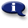# .

## Syllabus Information

Summer 2022
Sep 30,2023Use this page to maintain syllabus information, learning objectives, required materials, and technical requirements for the course.

Syllabus Information
MTH 111 - College Algebra
Associated Term: Summer 2022
Learning Objectives: Upon successful completion of this course, the student should be able to: 1. Maintain, use, and expand the skills and concepts learned in previous mathematics courses. 2. Apply the midpoint formula, distance formula, properties of lines, and equations of circles to the solution of problems from coordinate geometry. 3. Use and apply the concepts, language, notation, and evaluation of functions, including input-output ideas, domain, range, increasing, decreasing, maximum values, minimum values, symmetry, odd, even, composition of functions, and inverses. 4. Use substitution to create an equation defining one quantity as a function of another. 5. Apply principles of transformations (shifts, reflections, and stretches) to equations and graphs of functions. 6. Recognize, sketch, and interpret the graphs of the basic functions without the use of a calculator. 7. Identify and apply properties of polynomial functions. 8. Identify and apply properties of rational functions with and without a calculator. 9. Identify and apply properties of exponential and logarithmic expressions and functions. 10. Analyze a function by interpreting its graph, using a graphing calculator. 11. Translate a set of numerical data into graphical form, choose a function (linear or exponential) to model the data, and interpret the implications of the model (optional - time permitting). 12. Translate word problems into mathematical expressions, solve the problems, and interpret the solutions. 13. Communicate ideas of college algebra through English statements and mathematical sentences. 14. Use the language and skills of precalculus that are important for success in calculus. 15. Write and evaluate the notation of sequences and series including nth terms, summations, and factorials. 16. Solve nonlinear systems of equations algebraically and graphically (optional – time permitting). 17. Identify sequences as arithmetic, geometric, or neither and apply appropriate formulas related to those sequences to solve problems (optional - time permitting). 18. Accurately apply the mathematics learned in college algebra to topics from the student’s world.
Required Materials:
Technical Requirements: